# AWS - Arduino Weather Station

Very useful project made at school that detects some weather data.

BeginnerFull instructions provided25,793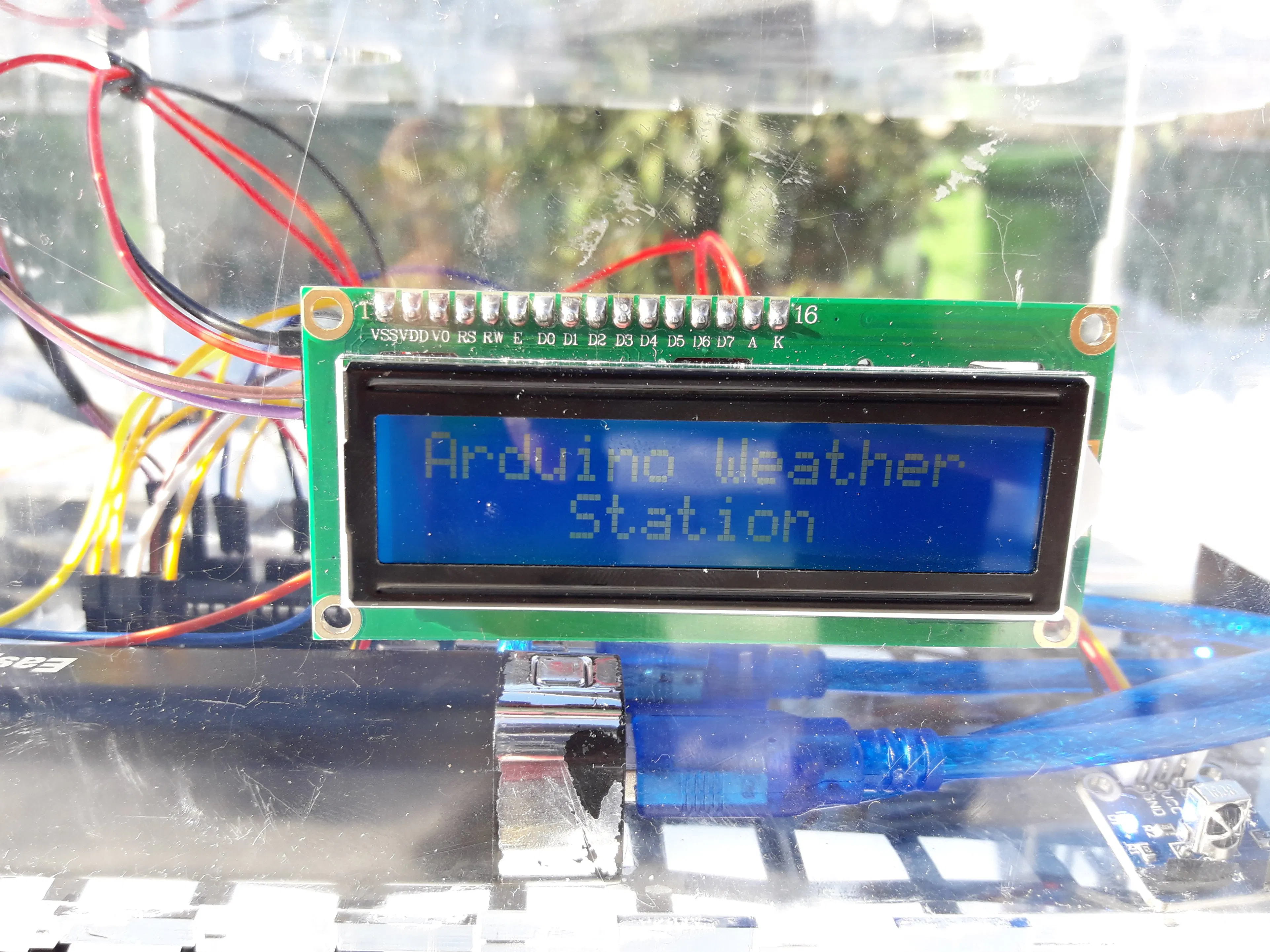## Things used in this project

### Hardware componentsArduino UNO & Genuino UNO
×1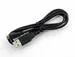USB-A to Micro-USB Cable
×1×1Jumper wires (generic)
×1
 Plexiglass 4 mm
×1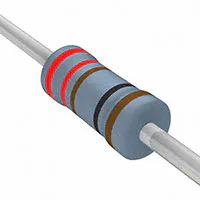Resistor 221 ohm
×1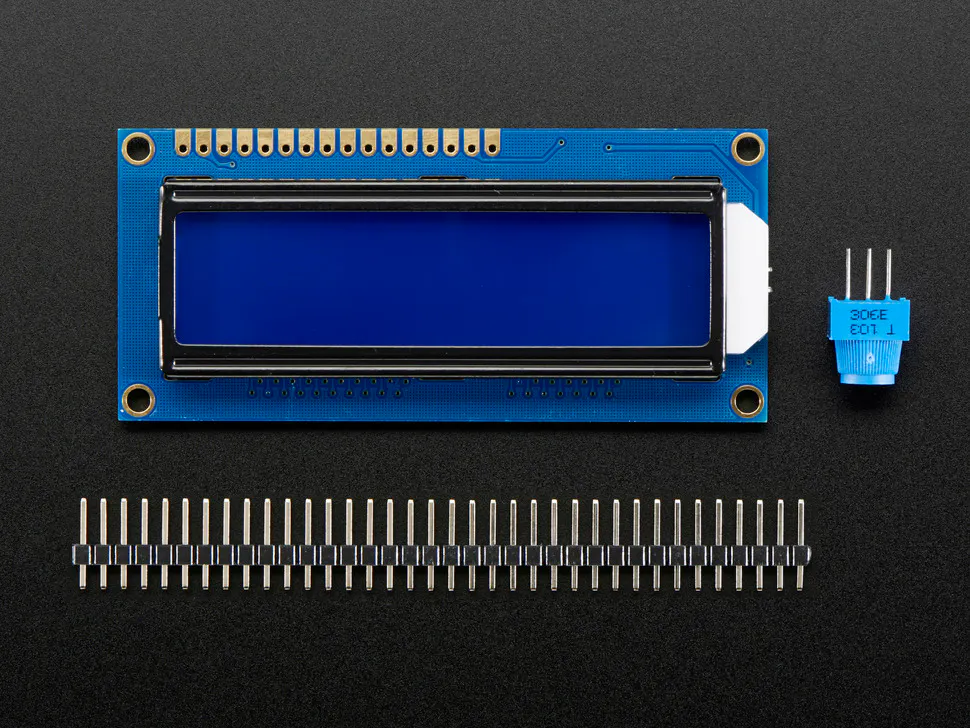Adafruit Standard LCD - 16x2 White on Blue
×1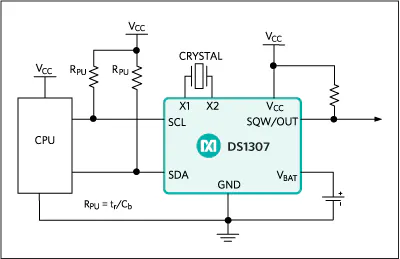Maxim Integrated DS1307 64 x 8, Serial, I²C Real-Time Clock
×1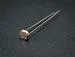Photo resistor
×1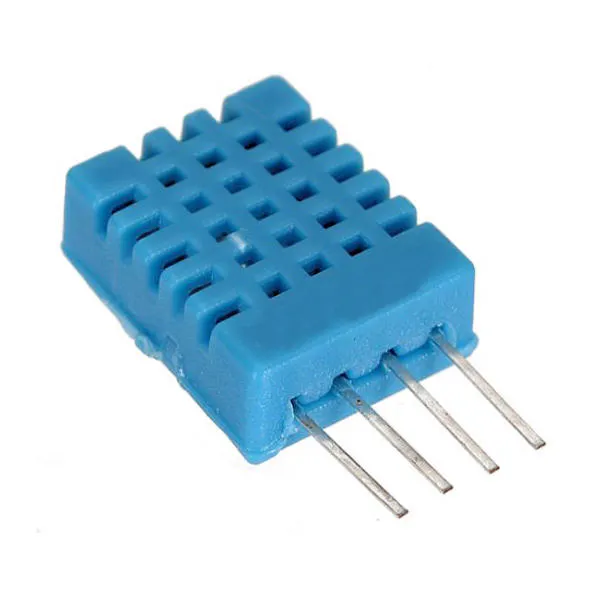DHT11 Temperature & Humidity Sensor (4 pins)
×1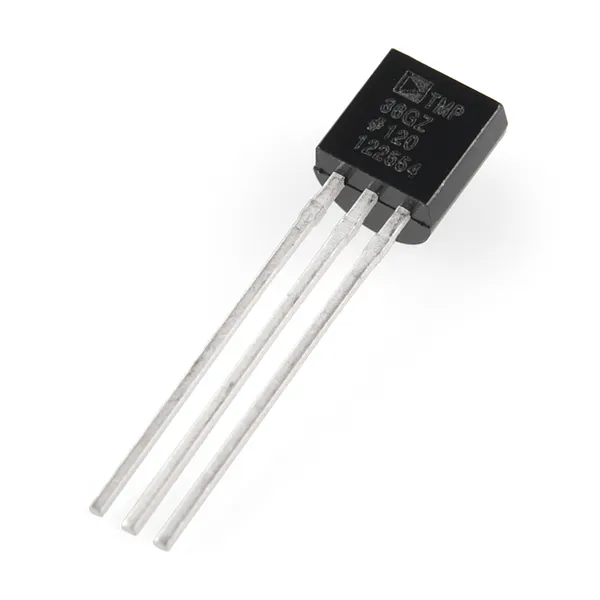Temperature Sensor
×1
×1
 Water Level Sensor
×1
 Sunfounder Starter RFID Kit
×1

### Hand tools and fabrication machines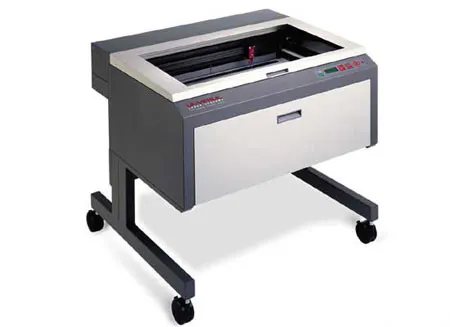Laser cutter (generic)

## Code

### AWS code.ino

C/C++
```//include sketch libraries
#include <IRremote.h>
#include <stdio.h> //clock library
#include <string.h> //clock library
#include <DS1302.h> //clock library
#include <dht.h> //dht11 library
#include <LiquidCrystal_I2C.h> //LCD library
#include <Wire.h> //Wire for LCD library

#define lmPin A1  //LM35 attach to A1

LiquidCrystal_I2C lcd(0x27, 16, 2); // set the LCD address to 0x27 for a 16 chars and 2 line display
dht DHT; //create a variable type of dht

const int DHT11_PIN = 4; //attach dht11 to pin 4
const int waterSensor = 0; //set water sensor variable
int waterValue = 0; //variable for water sensor
int mmwaterValue = 0;
int sensorPin = A3; // select the input pin for the potentiometer
int luce = 0; //variable for the ldr
int pluce = 0; //variable for the ldr
float tem = 0; //variable for the temperature
long lmVal = 0; //variable for the LM35
//ir
IRrecv irrecv(irReceiverPin);  //Creates a variable of type IRrecv
decode_results results;

//define clock variable
uint8_t RST_PIN   = 5;  //RST pin attach to
uint8_t SDA_PIN   = 6;  //IO pin attach to
uint8_t SCL_PIN = 7;  //clk Pin attach to
/* Create buffers */
char buf;
char day;

/* Create a DS1302 object */
DS1302 rtc(RST_PIN, SDA_PIN, SCL_PIN);//create a variable type of DS1302

void print_time()
{
/* Get the current time and date from the chip */
Time t = rtc.time();
/* Name the day of the week */
memset(day, 0, sizeof(day));
switch (t.day)
{
case 1:
strcpy(day, "Sun");
break;
case 2:
strcpy(day, "Mon");
break;
case 3:
strcpy(day, "Tue");
break;
case 4:
strcpy(day, "Wed");
break;
case 5:
strcpy(day, "Thu");
break;
case 6:
strcpy(day, "Fri");
break;
case 7:
strcpy(day, "Sat");
break;
}
/* Format the time and date and insert into the temporary buffer */
snprintf(buf, sizeof(buf), "%s %04d-%02d-%02d %02d:%02d:%02d", day, t.yr, t.mon, t.date, t.hr, t.min, t.sec);
/* Print the formatted string to serial so we can see the time */
Serial.println(buf);
lcd.setCursor(2, 0);
lcd.print(t.yr);
lcd.print("-");
lcd.print(t.mon / 10);
lcd.print(t.mon % 10);
lcd.print("-");
lcd.print(t.date / 10);
lcd.print(t.date % 10);
lcd.print(" ");
lcd.print(day);
lcd.setCursor(4, 1);
lcd.print(t.hr);
lcd.print(":");
lcd.print(t.min / 10);
lcd.print(t.min % 10);
lcd.print(":");
lcd.print(t.sec / 10);
lcd.print(t.sec % 10);
}

void setup() {
//clock
Serial.begin(9600);
rtc.write_protect(false);
rtc.halt(false);
//ir

lcd.init();  //initialize the lcd
lcd.backlight();  //open the backlight
pinMode(sensorPin, INPUT);
Time t(2017, 12, 9, 11, 20, 00, 7);//initialize the time
/* Set the time and date on the chip */
rtc.time(t);
}

void loop() {
lcd.setCursor(0, 0);
lcd.print("A");
delay(50);
lcd.setCursor(1, 0);
lcd.print("r");
delay(50);
lcd.setCursor(2, 0);
lcd.print("d");
delay(50);
lcd.setCursor(3, 0);
lcd.print("u");
delay(50);
lcd.setCursor(4, 0);
lcd.print("i");
delay(50);
lcd.setCursor(5, 0);
lcd.print("n");
delay(50);
lcd.setCursor(6, 0);
lcd.print("o");
delay(50);
lcd.setCursor(8, 0);
lcd.print("W");
delay(50);
lcd.setCursor(9, 0);
lcd.print("e");
delay(50);
lcd.setCursor(10, 0);
lcd.print("a");
delay(50);
lcd.setCursor(11, 0);
lcd.print("t");
delay(50);
lcd.setCursor(12, 0);
lcd.print("h");
delay(50);
lcd.setCursor(13, 0);
lcd.print("e");
delay(50);
lcd.setCursor(14, 0);
lcd.print("r");
delay(50);
lcd.setCursor(4, 1);
lcd.print("S");
delay(50);
lcd.setCursor(5, 1);
lcd.print("t");
delay(50);
lcd.setCursor(6, 1);
lcd.print("a");
delay(50);
lcd.setCursor(7, 1);
lcd.print("t");
delay(50);
lcd.setCursor(8, 1);
lcd.print("i");
delay(50);
lcd.setCursor(9, 1);
lcd.print("o");
delay(50);
lcd.setCursor(10, 1);
lcd.print("n");
delay(50);

{
if (results.value == 0xFF6897) //if "0" is pushed print TIME
{
lcd.clear(); //clear the LCD
print_time();
delay(10000); //delay 10000ms
lcd.clear(); //clear the LCD
delay (200); //wait for a while
irrecv.resume();    // Receive the next value
}
if (results.value == 0xFF30CF) //if "1" is pushed print TEMPERATURE and HUMIDITY
{
lcd.clear(); //clear the LCD
// DISPLAY DATA
lcd.setCursor(0, 0);
lcd.print("Tem:");
tem = (lmVal * 0.0048828125 * 100);//5/1024=0.0048828125;1000/10=100
lcd.print(tem);//print tem
lcd.print(char(223));//print the unit"  "
lcd.print("C      ");
// Serial.println(" C");
lcd.setCursor(0, 1);
lcd.print("Hum:");
//Serial.print("Hum:");
lcd.print(DHT.humidity, 1); //print the humidity on lcd
//Serial.print(DHT.humidity,1);
lcd.print(" %      ");
//Serial.println(" %");
delay(10000); //wait for 3000 ms
lcd.clear(); //clear the LCD
delay(200); //wait for a while
irrecv.resume();    // Receive the next value
}
if (results.value == 0xFF18E7) //if "2" is pushed print the DARKNESS
{
lcd.clear(); //clear the LCD
lcd.setCursor(4, 0); //place the cursor on 4 column, 1 row
lcd.print("Darkness:");
pluce = map(luce, 0, 1023, 0, 100); //the value of the sensor is converted into values from 0 to 100
lcd.setCursor(6, 1); //place the cursor on the middle of the LCD
lcd.print(pluce); //print the percentual
lcd.print("%"); //print the symbol
delay(10000); //delay 10000 ms
lcd.clear(); //clear the LCD
delay(200); //wait for a while
irrecv.resume();    // Receive the next value
}
if (results.value == 0xFF7A85) //if "3" is pushed print the SNOW or WATER LEVEL
{
lcd.clear(); //clear the LCD
lcd.setCursor(0, 0); //place the cursor on 0 column, 1 row
lcd.print("Fluid level(mm):"); //print "Fluid level(mm):"
int waterValue = analogRead(waterSensor); // get water sensor value
lcd.setCursor(6, 1); //place cursor at 6 column,2 row
mmwaterValue = map(waterValue, 0, 1023, 0, 40);
lcd.print(mmwaterValue);  //value displayed on lcd
delay(10000); //delay 10000ms
lcd.clear(); //clear the LCD
delay(200);
irrecv.resume();    // Receive the next value
}

if (results.value == 0xFF9867) //if "PRESENTATION" is pushed print TIME, TEM and HUM, DARKNESS and S or W LEVEL one time
{
lcd.clear(); //clear the LCD
print_time();
delay(4000); //delay 10000ms
lcd.clear(); //clear the LCD
delay (200); //wait for a while

// DISPLAY DATA
lcd.setCursor(0, 0);
lcd.print("Tem:");
tem = (lmVal * 0.0048828125 * 100);//5/1024=0.0048828125;1000/10=100
lcd.print(tem);//print tem
lcd.print(char(223));//print the unit"  "
lcd.print("C      ");
// Serial.println(" C");
lcd.setCursor(0, 1);
lcd.print("Hum:");
//Serial.print("Hum:");
lcd.print(DHT.humidity, 1); //print the humidity on lcd
//Serial.print(DHT.humidity,1);
lcd.print(" %      ");
//Serial.println(" %");
delay(4000); //wait for 3000 ms
lcd.clear(); //clear the LCD
delay(200); //wait for a while

lcd.setCursor(4, 0); //place the cursor on 4 column, 1 row
lcd.print("Darkness:");
pluce = map(luce, 0, 1023, 0, 100); //the value of the sensor is converted into values from 0 to 100
lcd.setCursor(6, 1); //place the cursor on the middle of the LCD
lcd.print(pluce); //print the percentual
lcd.print("%"); //print the symbol
delay(4000); //delay 10000 ms
lcd.clear(); //clear the LCD
delay(200); //wait for a while

lcd.setCursor(0, 0); //place the cursor on 0 column, 1 row
lcd.print("Fluid level(mm):"); //print "Fluid level(mm):"
int waterValue = analogRead(waterSensor); // get water sensor value
lcd.setCursor(6, 1); //place cursor at 6 column,2 row
mmwaterValue = map(waterValue, 0, 1023, 0, 40);
lcd.print(mmwaterValue);  //value displayed on lcd
delay(4000); //delay 10000ms
lcd.clear(); //clear the LCD
delay(200);
irrecv.resume();    // Receive the next value
}
}
}
```

## Credits

### GilettaStefano

0 projects • 18 followers